# VLOOKUP with IF statement in Excel

The tutorial shows how to combine VLOOKUP and IF function together to v-lookup with if condition in Excel. You will also learn how to use IF ISNA VLOOKUP formulas to replace #N/A errors with your own text, zero or blank cell.

Whilst the VLOOKUP and IF functions are useful on their own, together they deliver even more valuable experiences. This tutorial implies that you remember the syntax of the two functions well, otherwise you may want to brush up on your knowledge by following the above links.

## Vlookup with If statement: return True/False, Yes/No, etc.

One of the most common scenarios when you combine If and Vlookup together is to compare the value returned by Vlookup with a sample value and return Yes / No or True / False as the result.

In most cases, the following generic formula would work nicely:

IF(VLOOKUP(…) = value, TRUE, FALSE)

Translated in plain English, the formula instructs Excel to return True if Vlookup is true (i.e. equal to the specified value). If Vlookup is false (not equal to the specified value), the formula returns False.

Below you will a find a few real-life uses of this IF Vlookup formula.

#### Example 1. Look up a specific value

Let's say, you have a list of items in column A and quantity in column B. You are creating a dashboard for your users and need a formula that would check the quantity for an item in E1 and inform the user whether the item is in stock or sold out.

You pull the quantity with a regular Vlookup with exact match formula like this:

`=VLOOKUP(E1,\$A\$2:\$B\$10,2,FALSE)`

Then, write an IF statement that compares Vlookup's result with zero, and returns "No" if it is equal to 0, "Yes" otherwise:

`=IF(VLOOKUP(E1,\$A\$2:\$B\$10,2,FALSE)=0,"No","Yes")`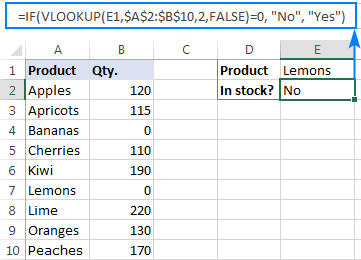Instead of Yes/No, you can return TRUE/FALSE or In Stock/Sold out or any other two choices. For example:

`=IF(VLOOKUP(E1,\$A\$2:\$B\$10,2)=0,"Sold out","In stock")`

You can also compare the value returned by Vlookup with sample text. In this case, be sure to enclose a text string in quotation marks, like this:

`=IF(VLOOKUP(E1,\$A\$2:\$B\$10,2)="sample text",TRUE,FALSE)`

#### Example 2. Compare Vlookup result with another cell

Another typical example of Vlookup with If condition in Excel is comparing the Vlookup output with a value in another cell. For example, we can check if it's greater than or equal to a number in cell G2:

`=IF(VLOOKUP(E1,\$A\$2:\$B\$10,2)>=G2,"Yes!","No")`

And here is our If formula with Vlookup in action: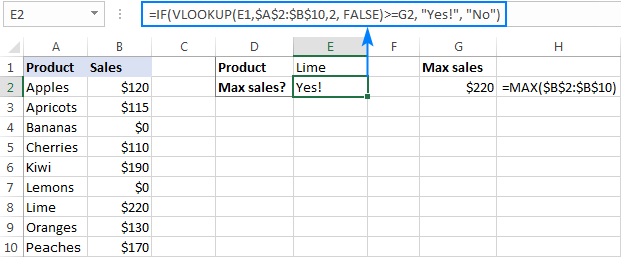In a similar fashion, you can use any other logical operator together with a cell reference in your Excel If Vlookup formula.

#### Example 3. Vlookup values in a shorter list

To compare each cell in the target column with another list and return True or Yes if a match is found, False or No otherwise, use this generic IF ISNA VLOOKUP formula:

IF(ISNA( VLOOKUP(…)),"No","Yes")

If Vlookup results in the #N/A error, the formula returns "No", meaning the lookup value is not found in the lookup list. If the match is found, "Yes" is returned. For example:

`=IF(ISNA(VLOOKUP(A2,\$D\$2:\$D\$4,1,FALSE)),"No","Yes")`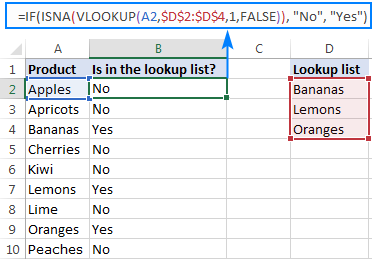If your business logic requires the opposite results, simply swap "Yes" and "No" to reverse the formula's logic:

`=IF(ISNA(VLOOKUP(A2,\$D\$2:\$D\$4,1,FALSE)),"Yes","No")`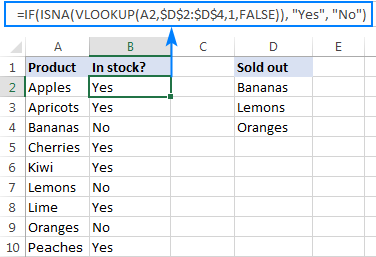## Excel If Vlookup formula to perform different calculations

Besides displaying your own text messages, If function with Vlookup can perform different calculations based on the criteria you specify.

Taking our example further, let's calculate the commission of a specific seller (F1) depending on their effectiveness: 20% commission for those who made \$200 and more, 10% for everyone else.

For this, you check if the value returned by Vlookup is greater than or equal to 200, and if it is, multiply it by 20%, otherwise by 10%:

`=IF(VLOOKUP(F1,\$A\$2:\$C\$10,3,FALSE )>=200, VLOOKUP(F1,\$A\$2:\$C\$10,3,FALSE)*20%, VLOOKUP(F1,\$A\$2:\$C\$10,3,FALSE)*10%)`

Where A2:A10 are seller names and C2:C10 are sales.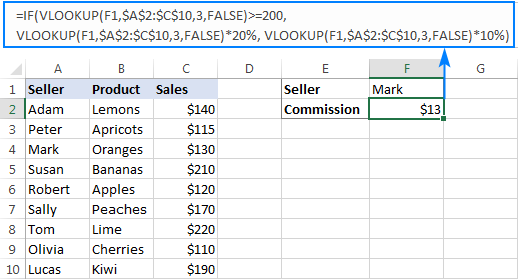## IF ISNA VLOOKUP to hide #N/A errors

If the VLOOKUP function cannot find a specified value, it throws an #N/A error. To catch that error and replace it with your own text, embed a Vlookup formula in the logical test of the IF function, like this:

Supposing, you have a list of seller names in one column and sales amounts in another column. Your task is to pull a number corresponding to the name the user enters in F1. If the name is not found, display a message indicating so.

With the names in A2:A10 and amounts C2:C10, the task can be fulfilled with the following If Vlookup formula:

`=IF(ISNA(VLOOKUP(F1,\$A\$2:\$C\$10,3,FALSE)), "Not found", VLOOKUP(F1,\$A\$2:\$C\$10,3,FALSE))`

If the name is found, a corresponding sales amount is returned: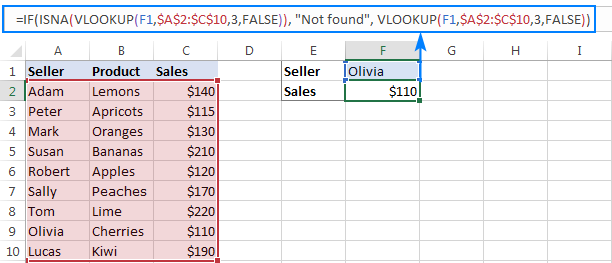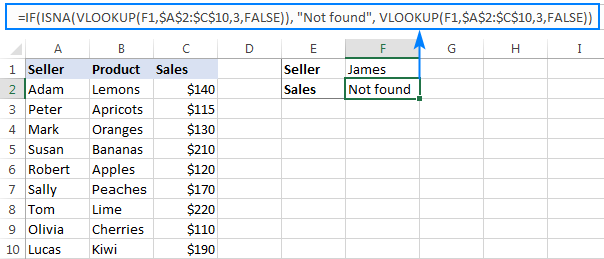#### How this formula works

The formula's logic is very simple: you use the ISNA function to check Vlookup for #N/A errors. If an error occurs, ISNA returns TRUE, otherwise FALSE. The above values go to the logical test of the IF function, which does one of the following:

• If the logical test is TRUE (#N/A error), your message is displayed.
• If the logical test is FALSE (lookup value is found), Vlookup returns a match normally.

## IFNA VLOOKUP in newer Excel versions

Beginning with Excel 2013, you can use the IFNA function instead of IF ISNA to catch and handle #N/A errors:

In our example, the formula would take the following shape:

`=IFNA(VLOOKUP(F1,\$A\$2:\$C\$10,3, FALSE), "Not found")`

Tip. If you'd like to trap all sorts of errors, not only #N/A, use VLOOKUP in combination with the IFERROR function. More details can be found here: IFERROR VLOOKUP in Excel.

When working with numerical values, you may want to return a zero when the lookup value is not found. To have it done, use the IF ISNA VLOOKUP formula discussed above with a little modification: instead of a text message, supply 0 in the value_if_true argument of the IF function:

IF(ISNA(VLOOKUP(…)), 0, VLOOKUP(…))

In our sample table, the formula would go as follows:

`=IF(ISNA(VLOOKUP(F2,\$A\$2:\$C\$10,3,FALSE)), 0, VLOOKUP(F2,\$A\$2:\$C\$10,3,FALSE))`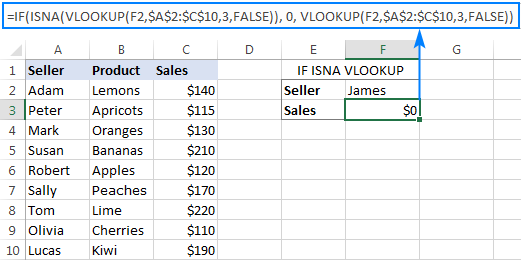In the recent versions of Excel 2016 and 2013, you can use the IFNA Vlookup combination again:

`=IFNA(VLOOKUP(I2,\$A\$2:\$C\$10,3, FALSE), 0)`

This is one more variation of the "Vlookup if then" statement: return nothing when the lookup value is not found. To do this, instruct your formula to return an empty string ("") instead of the #N/A error:

IF(ISNA(VLOOKUP(…)), "", VLOOKUP(…))

Below are a couple of complete formula examples:

For all Excel versions:

`=IF(ISNA(VLOOKUP(F2,\$A\$2:\$C\$10,3,FALSE)), "", VLOOKUP(F2,\$A\$2:\$C\$10,3,FALSE))`

For Excel 2016 and Excel 2013:

`=IFNA(VLOOKUP(F2,\$A\$2:\$C\$10,3, FALSE), "")`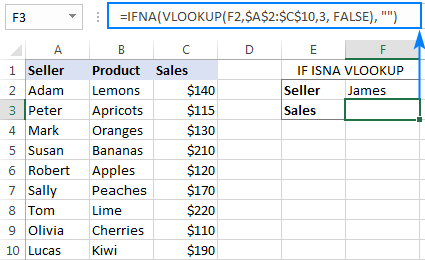## If with Index Match - left vlookup with If condition

Experienced Excel users know that the VLOOKUP function is not the only way to do vertical lookup in Excel. The INDEX MATCH combination can also be used for this purpose and it's even more powerful and versatile. The good news is that Index Match can work together with IF in exactly the same way as Vlookup.

For example, you have order numbers in column A and seller names in column B. You are looking for a formula to pull the order number for a specific seller.

Vlookup cannot be used in this case because it cannot search from right to left. Index Match will work without a hitch as long as the lookup value is found in the lookup column. If not, a #N/A error will show up. To replace the standard error notation with your own text, nest Index Match inside IF ISNA:

`=IF(ISNA(INDEX(A2:A10, MATCH(F1, \$B\$2:\$B\$10, 0))), "Not found", INDEX(A2:A10, MATCH(F1, \$B\$2:\$B\$10, 0)))`

In Excel 2016 and 2016, you can use IFNA instead of IF ISNA to make the formula more compact:

`=IFNA(INDEX(A2:A10, MATCH(F1, \$B\$2:\$B\$10, 0)), "Not found")`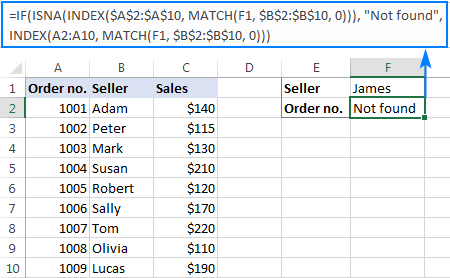In a similar manner, you can use Index Match in other If formulas.

This is how you use Vlookup and IF statement together in Excel. To have a closer look at the formulas discussed in this tutorial, you are welcome to download our sample workbook below. I thank you for reading and hope to see you on our blog next week!

Excel IF Vlookup - formula examples (.xlsx file)

## You may also be interested in

1. =IFERROR(IF(VLOOKUP(B35,\$S\$3:\$AB\$209,10,FALSE)=K35,"",=VLOOKUP(B35,\$S\$3:\$AB\$209,10),"")

Im in Row 35 because this was an example of Vlookup return false. I want the code to if false insert the value from the 10th column of the vlookup section

(I am using two sections of data to compare dates, if the date has updated it will return false which I currently have marked "check" and then I have to manually go back and check what the date was updated to in the database. The new date is already listed in the 2nd section of data but I want it to automatically populate the cell with the date instead of searching manually.)

The current formula doesnt work but I was hoping it would show what I am looking for.

• I can't understand your formula and check it as I don't have your data. I can't guess what result you wanted to get.

• I want a return false to display the value which is listed in the 10th row of the searched section

• If error, leave it blank. If true, leave it blank. If false show new value.

• Got it figured out

=IFERROR(IF(VLOOKUP(B35,\$S\$3:\$AB\$209,10,FALSE)=K35,"",VLOOKUP(B35,\$S\$3:\$AB\$209,10,False)),"")

For anyone needing a code like this

If Error Blank
If true Blank
If false display new value.

2. I don’t know if this is quite what I’m needing (probably far more advanced for me). I have 2 inventory sheets, Sheet1 with running totals of inventory incl column with prices. Sheet 2 is inventory taken by various sub trades. I’m trying to have sheet 2 calculate the individual costs per item taken on a specific entry. For simple example:
Sheet1 Totals Sheet 2
Item # Quantity Cost Item Quantity Job# Cost Date
1234 10 1.25. 2345 2 111 3.00. 7/18
2345 10 1.50. 1234 3. 121 3.75. 7/18
3456 10 1.75

Maybe ill answer my own thought, but I need Sheet 2 to input column D with IF A2 range is (Sheet1A2:A3)*(sheet1C2:C3) multiplied whatever the quantity is in Sheet 2 B2.

Is this even possible? I have about 150 items and would hate to have to put criteria for each individual item.

• Hi! Unfortunately, I didn't quite understand the problem. Maybe this article will be useful: Excel INDEX MATCH with multiple criteria. If not, please explain in more detail, give an example of the result you want to get.

3. I am trying to pull data based on multiple criteria. I have tier levels numbered 1,2,3, etc. Each tier level has amount in another column. Each customer has between 1 & 7 tiers. I need to lookup which amount makes up that tier level for each customer. (ie; customer #1 is currently at tier 2, what is the amount associated with tier 2 for that customer #).

Example:
Customer # Tier Level Amount
1 1 \$0
2 \$1,000
3 \$2,000

Thanks!! :)

• Thanks! That is close, but I need to reference a different cell for the value and they are on multiple sheets as well. I am not able to paste a screenshot so hopefully you can decipher this. ;)

Sheet 1 - Column A has the customer number (each customer number has 1 row), Column I is where I need to add the formula to find the Lower Tier Amount for that customer.

A H I
Cust Lower Tier Rank Lower Tier Min
61523 - ??? (needs to return 0)

Sheet 2 - Column A has the customer number (each customer number has 1-7 rows of tier levels), Column I is the Lower Tier Amount, Column Q shows the current lower tier number (ie, tier 1, tier 2).

A I J P Q R
Cust Volume From Volume To Current Tier Lower Tier Higher Tier
61523 0 700,000 1 - 2
61523 700,000 1,000,000 0 (1) 1
61523 1,000,000 999,999,999,999,999 0 (1) 1

Thanks!!! :)

• Sorry, I knew it would mess up that formatting. A H I & A I J P Q R are the columns and the data for each column is listed below it (but bunched together).
Sheet 1 - Column A - Cust, (61523) Column H - Lower Tier Rank (-), Column I - Lower Tier Min (??? this is the value I need - should return a 0). Sheet 2 - Column A - Cust, (61523), Column I - Volume From (0), Column J - Volume To (700,000), Column P - Current Tier (1), Column Q - Lower Tier (-), Column R - Higher Tier (2).

• On sheet one Column I is the amount for the tier that is in Q (this is the value I need returned on sheet 2). Sheet one has; Customer # in column A, and their tier level number on column Q.

On sheet 2, Column A has the customer number. Column H has the tier level number.

I need sheet 2, column I to tell me what the amount is on sheet 1 that matches both the customer number and the tier number.

Sorry, I wish I could add screenshots. :)

• If I understand correctly, study this manual carefully: Excel INDEX MATCH with multiple criteria. Try a formula like this:

=INDEX(Sheet2!I2:I100, MATCH(1,(Sheet1!A2=Sheet2!A2:A100)*(Sheet1!Q2=Sheet2!H2:H100),0))

• That is exactly what I needed! Thank you so much for all your help!! :)

• Sorry, it's not quite clear what you are trying to achieve.

4. Hello
i have 12 seet of 12 month and i want to make one master seet all seet have pary name and its payble amount , for exampale , we have to do 2000 payment to abcd party in jan. And 3000 in feb. How can i make master seet to seet all month payment in row, i want formula that put jan paybale ammount in jan cell ,not in diffrent month cell .
Thank you.

5. I have two Columns:

Column A Column B

OLF2-09-AI-001 1"-AI-130486-BAE3-IS50
OLF2-09-AI-003 1/2"-AI-130586-A7A
OLF2-09-AI-005 12"-AI-137584-AR3-IH25
OLF2-09-BH-001 1"-BH-130486-A7A
OLF2-09-BH-001 12"-BH-135846-A7A
OLF2-09-BH-001 8"-BH-135874-A7A
OLF2-09-BH-001 14"-BH-132145-A7A
OLF2-09-GR-001 1"-GR1358100-AS3-IC
OLF2-09-GR-001 1"-GR-130004-A7A
OLF2-09-GR-001 1"-GR-130005-A7A
OLF2-09-GR-001 1"-GR-1300001-A7A
OLF2-09-NHD-004 1"-GR-136666-A7A

I want to get result as value in column B against similar value of column A with largest number with " sign.

• Hi! From your description, I can't understand what result you want to get. To search for the largest number, extract it from the text using the LEFT function and SEARCH function
Try this formula:

=LEFT(B1,SEARCH("""",B1)-1)

6. Hi I am hoping to use excel to drive an advice action

Column 2 = drop down list
Column 3 = will return a text based action based on the response in Column 2

For example:
drop down = yes => Talk to Mel
drop down = no => leave blank
drop down = not sure => Talk to Sam
drop down = [any other choice] => Further information required

7. Hi, I have cell A1 Apples and Cell A2 Lemons on Sheet 2. Cell B1 and 2 is qty, now i have Cell A3 Apples & Lemons and Cell B3 qty. On my next Sheet
I have Cell A1 Apples and Cell A2 Lemons. I want to vlookup the second sheet and count the qty over to Sheet 1, prob is i have Cell A3 with Apples and Lemons, but need to split count to sheet 1 ex: Apples and Lemons in Cell A3 qty is 4, now this must count to sheet 1 as apples 2 and lemons 2 and or apples =4 and lemons =4, either one of the 2 solutions will work as i have a database working with machinery. On my excel apples will be a machine (DD211) and lemons will be (DD2710) they use the same parts therefore sometimes I get 1 order for both machines, I still need separated data as to what was ordered for each, So it should be order 1 for DD2710 and DD211, 50 parts, on sheet 2 it must show DD211 1 order Cell A1 and cell A2 DD2710 1 order or it could shou DD211 25 parts Cell A1 and 25 Parts DD2710 cell A2, Hope i explained enough detail

Thank you

• Hi!
The VLOOKUP function cannot search for a match over a part of the text in a cell. Also, your problem is very confusing and cannot be solved by a single formula. If you have a specific question about the operation of a function or formula, I will try to answer it.

8. I want to lookup and the "where to look up" is in a cell among other values. How should be the formula in this case?

For example, I have my data with A,B,C,D,E, I want to know for those a specific information linked, but sometimes the cells could be filled like A ; B ; C or A ; B or A : E.

The "where to look up value" is then not always alone in its cell like "A" to do the proper formula.
Hope it is clear enough :)

• Hi!
You can use the SEARCH function to search for a value in a text string in a cell. I'm sorry, I'm afraid these pieces of info are not enough to give you a formula.

9. 1) For Book A, I have 5 copies, For Book B, I have 10 copies, For Book C, I have 15 copies. I have 1000 books with different titles & quantity.
2) I have to distribute these copies to different schools & also simultaneously maintain how many copies I have given to whom & how many are left.

10. Hi!
I have 6 columns.
In first one - A i have a list of shop names, in the second one B I have code of selled products, and on the sixth column F i have quantity of this selled products:

Table1:
A B F
Shop 1 Product 1 Quantity 1 (5)
Shop 2 Product 1 Quantity 2 (4)
Shop 3 Product 1 Quantity 3 (0)
Shop 4 Product 1 Quantity 4 (3)

In other table i need to extract from this first table for example The Quantity that Shop 3 is selled and to put it in exact cell.

Table2:
A B
Shop 3
Product 1 Quantity 3

I tried with combination of VLOOKUP and IF, but withot the result i need...

Thank you!!!

11. I need to do vlookup only if date for same lookup value in one sheet is greater than date of that return value in lookup array in another sheet. what is formula for this please.

• Hi!
I'm sorry, I'm afraid these pieces of info are not enough to give you a formula.

12. hey magic excel guys,

Scenario: part number "x" & "y" have different locations and each location has its own quantity of x & y. see below.

Item No Location Qty
x A 5
B 4
y A 3
D 12

I need to be able to lookup value x only in location A to get the quantity. (my excel sheet has 3804 rows of data similar to the above)

Here's the formula i came up with:

=IF(VLOOKUP(A1,table_array,col_index_num,false)=A, (A1,table_array,col_index_num,false), "Not available")

In my mind, this makes sense, but excel thinks otherwise.

Grateful for any help here.

Cheers

13. Hi, I am searching for a number in the other sheet and I want the answer in "Y" or "n". I will explain this in detail,
For ex. if I enter anything in D4 and want to know whether that number is present in the other sheet or not. And I want that results in column V4 with the colour Green for "Y" and Red for "N".

How can I make a formula for the above requirement as I have tried it using IF(IFERROR(VLOOKUP)) functions, but something is wrong.

• Hi!
The conditional formatting formula for cell V4 might look like this:

=ISERROR(VLOOKUP(D4, Sheet2!\$A\$2:\$B\$6, 2, FALSE)) for Red
=NOT(ISERROR(VLOOKUP(D4, Sheet2!\$A\$2:\$B\$6, 2, FALSE))) for Green

• Actually still I did not got the proper solution, Currently I have been using the below formula for the above condition

=+IF(D4="","",IFERROR(VLOOKUP(TEXT(D4,"0000000"),[LIST_aktuell_GesamtbestandCTeile_V1_AR2222.xlsx]Tabelle1!\$B\$4:\$O\$30000,2,FALSE),"N"))

LIST_aktuelle_....is the sheet name.

Can you correct if you have understood the problem.

I have already done the conditional formatting for the Yes or No.

• Hi!
I don't quite understand what result you want to get. I can't see your data so I can't check the formula. Note that you are looking for text. The search data must also be text.

• Hi,
If I put any Material number (which includes text and numbers) in A2 and then I want to know whether the material number which I am searching for is present in the other sheet or not by the result of "Y"OR "N" in the field V2.

I know its hard to understand this without seeing the data, but just wanted to know which formulas I can use.

14. Hi, can somebody help me please.. can someone give me a formula wherein.. "if I input any any TEXT on C1, "latest date will show in B1" and "number will show in A1". And a formula wherein "if I input TEXT in C5", it will also show the other values beside it in B and A column.

Thank you so very much and God bless you.

• Hi!

• use this formula

in B1
=IF(ISTEXT(C1);"latest date";"")

in A1
=IF(ISTEXT(C1);"number";"")

15. Hi, I would like a formula for the below criteria

I have two sheets, the first fixed one contains the restaurant names with their fixed delivery charge in the column next to them, E.g Restaurant A (delivery charge 10), Restaurant B (delivery charge is 12), etc..

The second sheet will contain the deliveries done for the month. So if the name of the restaurant matches its equivelant name in the first sheet, then the delivery charge is 10. If the second row also has restaurant A then charge is 10 again, if third row contains restaurant B, then delivery charge is 12.

Thank you

16. I want to have a function that will check the rows. Columns A to C can contain a value or word "Dummy". In Column D, I want to return the values that is not the word "dummy".

• Hi!
I don't know how you would like to get the result. For example:

=TEXTJOIN(",",TRUE,IF(A1:C1="Dummy","",A1:C1))

TEXTJOIN function concatenate cell values with a delimiter.

17. Hi,

I am trying to add a condition to the following VLOOKUP:
=IF(A25="","",IF(A250,VLOOKUP(A25,'Product List'!\$A\$2:\$H\$73,8,FALSE)))

The condition I would like to add, is if cell E25=Lemons then =IF(A25="","",IF(A250,VLOOKUP(A25,'Product List'!\$A\$2:\$H\$73,8,FALSE)))

So that the =IF(A25="","",IF(A250,VLOOKUP(A25,'Product List'!\$A\$2:\$H\$73,8,FALSE))) only works if E25 has Lemons entered in the cell. Is this even possible?

Thank you for your help. I have been trying to solve this all day (I am not an advanced Excel user). :(

• Hello!
If I understand correctly, you can add another nested IF function.

=IF(E25="Lemons", IF(A25="","",IF(A250,VLOOKUP(A25,'Product List'!\$A\$2:\$H\$73,8,FALSE))),"")

18. suppose x employee OT done in jan-22 & claim in feb-22, again in mar-22 try to claim ot agst jan-22, then how to track/stop claim twice/double OT date in which excel formula to use

• Hi!
I am not sure I fully understand what you mean.

19. I would need help on the following conditions please if possible.

If Column A meet the criteria and Column B meet another criteria, return Column C.
But if the Column C is blank, look for the next return value under Column C where Column A & B still both meets the criteria.

20. Hi, I need exactly what describe in the scenario of "Vlookup with If statement: return True/False, Yes/No, etc." With only one twist.
Imagine I have multiple rows of "Lemons" in column A with different values (10,6,etc) and I need the function to tell me true/false only if one of the "Lemon" rows hits the value of "0" value in column B ignoring the others (10,6,etc).
Is that possible?

21. =IF(AE3=\$AQ\$2,IF(AC3=\$AR\$2,IF(AI3=\$AS\$2,IF(AJ3=\$AT\$2,VLOOKUP(B3,B3:AM422,3,FALSE)))))
I am trying to a command that says IF(\$AQ\$2=N/A, false, otherwise give me the value).
I was using this formula to pull data from a large data base using a drop down list. However, It is requiring me to choose all 4 criteria's, otherwise, it gives me the false result instead of giving me a number for one and false for the other.

Let's say I have this headers for a drop down list: State City Tenancy (Elderly, Family, and Other) Construction Type (New, Rehab)

Depending on the criteria's above, I commanded it to If(AE3-\$AQ\$2....VLOOKUP ((B3,B3:AM422,3,FALSE)) \$AQ\$2 is the state and such. AE3 is the criteria from the big data base.

Depending on my criteria choice, i wanted it to give me the values for the different expenses i was looking for: Management fees, utilities, etc.

The bottom line of my problem is that If I want to see all the properties in Indiana, with a tenancy type of Family and construction type of Rehab, and I don't care about which cites these projects are located so I select N/A, it gives me false for all criteria's instead of values for the criteria's I choose. How can I command it so that if I selected the stat, the tenancy Type, and the the construction type, it will give me the appropriate values. But since I don't want the city this time, I am trying to command it if City is "N/A), then give me false.

• Hello!
If I understood your problem correctly, you want to define a N/A error in a cell. Try using the ISNA function for this.
IF(ISNA(\$AQ\$2), false, otherwise give me the value)
Hope this is what you need.

22. I am trying to write a formula where if a cell = EBAY then take item number in column B and vlookup a range of data. Any ides?

• Hi!
The information you provided is not enough to understand your case and give you any advice, sorry. Please describe your problem in more detail.

23. Hello - I am trying to verify if a delivery time was within the scheduled delivery window, can someone help with a formula?

Arrival Time Departure Time Start Time End Time DELIVERED DURING CORRECT TIME SLOT
8/1/2022 2:28:16 AM 8/1/2022 3:01:45 AM 0 300 TRUE

• Hello!
To determine if the time is in the correct interval, use the IF AND formula:

=IF(AND(C1>A1,C1<B1),TRUE,"")

24. Great article,

I probably am missing something easy, but how can I use
=IF('sales-breakdown-jul1-12-2022 '!A:A=A3,VLOOKUP("Liquor",'sales-breakdown-jul1-12-2022 '!\$B:\$D,2,FALSE))
where A3 is a cell within a name bank. When I write the person's name in "John Doe" into the logical test, it works but I don't want to write a formula for each new person each time we run report.

• Hi!
Try to replace the text in the formula with a cell reference. Write in this cell the desired text. I hope it’ll be helpful.

25. Hello Alex,

Thank you for this great article. I only came to know of ISNA & IFNA after i read this. I have been struggling with this formula since morning as the formula works in some cells and shows N/A in some cells. I have a sheet that has to look up the country, if US, then check if it is a corporate or client , look up the pay range in the adjacent cell then give a value, If not US, there is no differentiation and picks the defined range for non-US. What bothers me is when i correct the formula by doing the single v-look up in the N/A cell, the value pops up, but when I drag the formula its a ruin.

Could you guide me on what I should be doing.

=IF(P11="United States of America",IF(AB11="Corporate",VLOOKUP(H11,'Main Pay Ranges'!Q11:R22,2,0),VLOOKUP(H11,'Main Pay Ranges'!E11:F22,2,0)),VLOOKUP(H11,'Main Pay Ranges'!E45:F57,2,0))

• Hi!
I can't check the formula that contains unique references to your workbook worksheets, sorry.
I think you need to use absolute references for search ranges: VLOOKUP(H11,’Main Pay Ranges’!\$Q\$11:\$R\$22,2,0)
Maybe it will help.

26. Hi
in BS 2 value is 1 and i am trying to use below formula.

=IF(BS2="1",(VLOOKUP(BG2,Sheet1!\$F\$6:\$G\$67,2,0)))

but its still showing " False" in cell .

Can you help me with this.

Thanks you.

27. I have created a dropdown menu y/n. If yes, then the formula should get a value in another sheet and if no, it should just be a 0.
Yes/No - If yes, pick up value in cell x; if no = 0 - I have tried several ways. Someone that might have an idea?
Thanks.

• Hello!
If your drop-down menu inserts a value in cell A1 then you can use IF function:

=IF(A1="Yes",X1,0)

Hope this is what you need.

28. Can you help with with what my formula format would be if I want to pull a cost from another tab if certain criteria matches in both tables?
Ie. I have a master inventory list that has serial numbers costs, etc. When I enter the sale of that serial number on another tab I want it to auto populate the cost associated with that serial entered on the inventory tab. Is that possible?

Thank you

29. Hello, I am hoping you can steer me in the right direction. I have a spreadsheet for tracking monthly hours. It has a sheet for each month and a Summary sheet, which should show the sum of each person's hours for the year. There is a unique identifier (employee number) for each employee, so I set up a formula using SUM and VLOOKUP in the Summary sheet. I named a Range on each spreadsheet (jan, feb, mar, etc.), so it looked something like this (I am still experimenting, so the formula only goes to May):
=SUM(VLOOKUP(A2,jan,3,FALSE)+VLOOKUP(A2,feb,3,FALSE)+VLOOKUP(A2,mar,3,FALSE)+VLOOKUP(A2,apr,3,FALSE)+VLOOKUP(A2,may,3,FALSE))

The hitch: we start with a certain number of employees in the spring, and hire seasonal workers throughout the year. So the list of names in January might be 400, by April it might be 650, and by August 800. My formula works fine for the first 400 folks, but when I add more names to the spreadsheets for the following months (and extend the Ranges to include the new names), the Summary results come up as N/A for the new names. If I add an IFNA function, all new names after the first spreadsheet (jan) bring up "0" in the Summary instead of N/A:
=SUM(IFNA(VLOOKUP(A2,jan,3,FALSE)+VLOOKUP(A2,feb,3,FALSE)+VLOOKUP(A2,mar,3,FALSE)+VLOOKUP(A2,apr,3,FALSE)+VLOOKUP(A2,may,3,FALSE),0))

I am still very much an Excel novice, so any assistance would be very appreciated!

• Hello!
I can't check the formula that contains unique references to your workbook worksheets. However, you are using the IFNA function incorrectly. Try to change the formula:

=SUM(IFNA(VLOOKUP(A2,jan,3,FALSE),0)+IFNA(VLOOKUP(A2,feb,3,FALSE),0)+IFNA(VLOOKUP(A2,mar,3,FALSE),0)+IFNA(VLOOKUP(A2,apr,3,FALSE),0)+IFNA(VLOOKUP(A2,may,3,FALSE),0))

• Thank you very much for your help - I am off to learn about Pivot Tables!

30. Hi,

I'm struggling to find the right formula to multiply units by rates.

I have different materials and tasks with different units and rates are depend on quantities. Some of the units only have one rate with no conditions.

I have a more than 2000 row spreadsheet and units also varies that means that the formula also need to find the unit on sheet 1. Rate criteria can also change on sheet 1.

I'm looking for the price on Sheet2.

I believe the below formula need to be combined with vlookup but I cannot get it to work

Niki

Sheet 1
Unit "Rate1(not exceeding)""Rate2(not exceeding)""Rate3(not exceeding)""Rate4(exceeding)"
day
h
m (QB) 10 50 200 200
m
m2 (QB) 10 50 200 150
m2

Sheet 2
Description Unit Quantity Rate 1 Rate 2 Rate 3 Rate 4 Price
path m (QB) 10 11 13 13.5 14
road m (QB) 51 5 10 15 20
wall m2 (QB) 35 10 15 20 25
wood m 20 11
paint m2 150 12

31. I have a dataset where a single person will have several records that look very similar except for the date column.

So if BRAD has 8 apples as of Jan 1 (row 1) and 10 apples as of March 10 (row 2) and 4 apples as of April 4 (row 3), I would want to return a value of 4 because it is the most recent date.

BRAD - APPLES - 1/1/2022 - 8
BRAD - APPLES - 3/10/2022 - 10
BRAD - APPLES - 4/4/2022 - 4

Is there a way to accomplish this with VLOOKUP and IF functions?

• Hello!
Search using INDEX+MATCH. You can find the examples and detailed instructions here: Excel INDEX MATCH with multiple criteria.

Hope this is what you need.

32. Hello! may ask something, about vlook up, how can I automatically add new text in the table, then automatically apply in the advanced filter?

33. Im creating a gsheet for prorated leave balance, if employee reach 2 yrs in service she/he will have 14 leave credits, if reached 3, 4, or 5 yrs will have 15 leave credits.

But if employee reached 1 year, it will be prorated. For example her one year fall on January 1 to 15, automatically she will have 12 leave credits but if her one year fall on January 16-feb 15, she will only have 11 credits, if one year fall on Feb 16-march15, she will have 10 credits and so on. How can I formulate that?

• Hello!
Count the number of months using the MONTH function -

=12-MONTH(A1)+(DAY(A1)<16)

34. A Cell - B Cell the answer is C cell. if B cell 0 Mean the Answer C Cell should get value for A Cell.

My question is If B Cell 0 Value mean should C Cell also need get value 0.

therefore, what is the function i need put in C Cell.